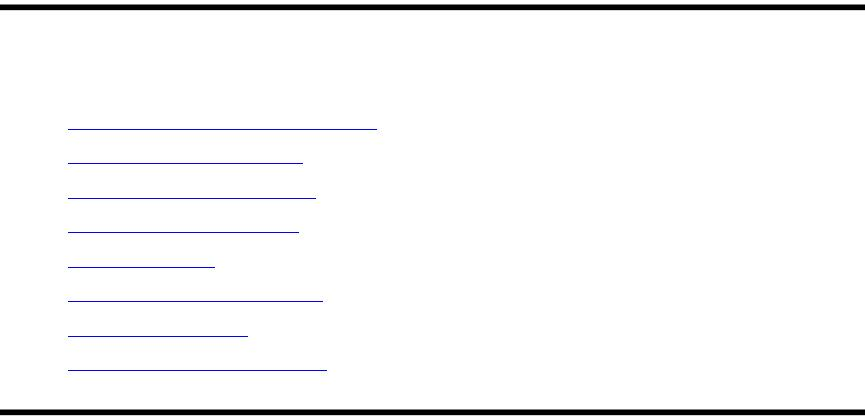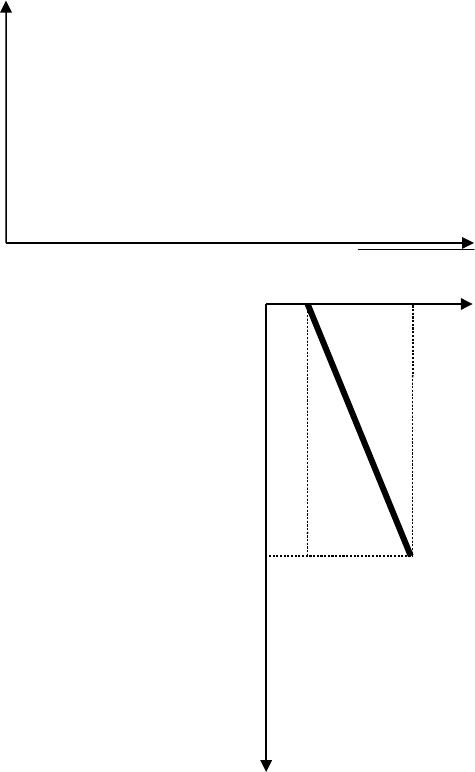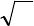Type
Solution Manual
Book Title
Fundamentals of Investments: Valuation and Management 8th Edition
ISBN 13
978-1259720697

### 978-1259720697 Chapter 13 Lecture Note

January 2, 2020
Chapter 13
Performance Evaluation and
Risk Management
Slides
13-1. Chapter 13
13-2. Performance Evaluation and Risk Management
13-3. Learnig Objectives
13-4. Performance Evaluation and Risk Management
13-5. Performance Evaluation Measures, I.
13-6. Performance Evaluation Measures, II.
13-7. Performance Evaluation Measures, III.
13-8. Performance Evaluation Measures, IV.
13-9. Jensen’s Alpha
13-10. Another Method to Calculate Alpha, I.
13-11. Another Method to Calculate Alpha, II.
13-12. Estimating Alpha Using Regression, I.
13-13. Estimating Alpha Using Regression, II.
13-14. The Information Ratio
13-15. R-Squared, I.
13-16. R-Squared, II.
13-17. Investment Performance Measurement on the Web
13-18. Comparing Three Well-Known Performance Measures: Be Careful
13-19. Comparing Performance Measures, I.
13-20. Comparing Performance Measures, II.
13-21. Global Investment Performance Standards
13-22. Sharpe-Optimal Portfolios, I.
13-23. Sharpe-Optimal Portfolios, II.
13-24. Sharpe-Optimal Portfolios, III.
13-25. Example: Solving for a Sharpe-Optimal Portfolio
13-26. Example: Using Excel to Solve for the Sharpe-Optimal Portfolio
13-27. Example: Using Excel to Solve for the Sharpe-Optimal Portfolio, Cont.
13-28. Investment Risk Management
13-29. Value-at-Risk (VaR)
13-30. Example: VaR Calculation, I.
13-31. Example: VaR Calculation, II.
13-32. Example: VaR Calculation, III.
13-33. Example: A Multiple Year VaR, I.
13-34. Example: A Multiple Year VaR, II.
13-35. Example: A Multiple Year VaR, III.
13-36. Computing Other VaRs
13-37. Useful Websites
13-38. Chapter Review
McGraw-Hill
Education.Performance Evaluation and Risk Management 13-2
Chapter Organization
13.1 Performance Evaluation
A. Performance Evaluation Measures
B. The Sharpe Ratio
C. The Treynor Ratio
D. Jensen’s Alpha
E. Another Method to Calculate Alpha
F. Information Ratio
G. R-Squared
13.2 Comparing Performance Measures
A. Global Investment Performance Standards
B. Sharpe-Optimal Portfolios
13.3 Investment Risk Management
A. Value-at-Risk
13.4 More on Computing Value-at-Risk
13.5 Summary and Conclusions
Selected Web Sites
www.stanford.edu/~wfsharpe (visit Professor Sharpe’s homepage)
www.morningstar.com (comprehensive source of investment information)
www.garp.org (The Global Association of Risk Professionals)
www.andreassteiner.net (Performance Analysis)
www.finplan.com (FinPlan – financial planning web site)
Annotated Chapter Outline
13.1 Performance Evaluation
Performance Evaluation: Concerns the assessment of how well a money
manager achieves a balance between high returns and acceptable risks.
McGraw-Hill
Education.Performance Evaluation and Risk Management 13-3
In general terms, performance evaluation focuses on assessing how well a
money manager (either a professional or an individual investor) achieves high
returns balanced with acceptable risks.
Performance evaluation is particularly significant when we consider efficient
markets. In a previous chapter, we raised the question of risk-adjusted
performance and whether anyone can consistently earn an "abnormal" return,
thereby "beating" the market. Our goal here, however, is to introduce the primary
assessment tools, rather than discuss whether we should entrust our investment
funds with fund managers.
A. Performance Evaluation Measures
Raw Return: States the total percentage return on an investment without
any adjustment for risk or comparison to any benchmark.
The raw return on a portfolio is a naive measure of performance evaluation. The
fact that a raw portfolio return does not reflect any consideration of risk suggests
that its usefulness is limited when making investment decisions.
So, we shall examine some of the best-known and most popular measures that
B. The Sharpe Ratio
Sharpe Ratio: Measures investment performance as the ratio of portfolio
risk premium over portfolio return standard deviation. This ratio was
originally proposed by Nobel Laureate William F. Sharpe.
p
fp
σ
RR
Ratio Sharpe
The portfolio risk premium, Rp – Rf, is the basic reward for bearing risk, while the
return standard deviation is a measure of the total risk for a security or a portfolio.
We referred to the risk premium as “excess return” in a previous chapter.
The Sharpe ratio is a reward-to-risk ratio that focuses on total risk. The
systematic risk principle states that the reward for bearing risk depends only on
the systematic risk of an investment. So no matter how much total risk an asset
has, only the systematic portion is relevant in determining the expected return
(and the risk premium) on that asset. Hence, the Sharpe ratio is probably most
appropriate for evaluating relatively diversified portfolios, since it penalizes non-
diversified portfolios by also taking into account unsystematic risk.
The Sharpe ratio uses total standard deviation, but investors are likely only
concerned about downside volatility (i.e., returns below expectation). The Sortino
McGraw-Hill
Education.Performance Evaluation and Risk Management 13-4
ratio is calculated just like the Sharpe ratio, but the deviation is calculated using
only returns that are below the mean. Thus, the Sortino ratio does not penalize
for upside volatility.
C. The Treynor Ratio
Treynor Ratio: Measures investment performance as the ratio of portfolio
risk premium over portfolio beta. Jack L. Treynor originally proposed this
measure.
p
fp
β
RR
Ratio Treynor
As with the Sharpe ratio, the Treynor ratio is a reward-to-risk ratio. The key
difference is that the Treynor ratio looks at systematic risk only, not total risk.
D. Jensen’s Alpha
Jensen's Alpha: Measures investment performance as the raw portfolio
return less the return predicted by the Capital Asset Pricing Model
(CAPM). This measure was originally proposed by Michael C. Jensen.
fMpfp RREβRRE:Return Expected Portfolio the CAPM, the From
fMpfpppp
RREβRRRERα Alpha,sJensen'
Jensen's alpha is simply the abnormal return above or below the security market
line. In this sense, it can be interpreted as a measure of how much the portfolio
"beat the market." A positive alpha is a good thing because the portfolio has a
relatively high return given its level of systematic risk.
E. Another Method to Calculate Alpha
Following the same approach as we used to find beta, alpha can be found using
a regression of the security returns (above the risk free rate) on the market or
index (excess return). The resulting intercept is an estimate of the alpha. One
advantage of this approach is that the regression output provides a measure of
the significance of this estimate.
F. Information Ratio
Information Ratio: A security’s alpha divided by its tracking error.
McGraw-Hill
Education.
Performance Evaluation and Risk Management 13-5
Tracking error is the deviation of the annual difference between the security’s
excess return and the market’s excess return. Dividing alpha by tracking error
essentially penalizes the fund manager for excessive volatility of returns.
G. R-squared
Squaring the correlation of the fund’s returns to the market’s returns gives R-
squared. This is the percentage of the fund’s returns that are driven by the
market. A higher R-squared gives confidence to the alpha estimate.
13.2 Comparing Performance Measures
As illustrated by the example in the text, the three basic performance measures
can yield substantially different performance rankings. So, which measure should
be used to evaluate portfolio performance? The simple answer is, "It depends."
To evaluate an entire portfolio held by an investor, the Sharpe ratio is
appropriate. To evaluate securities or portfolios for possible inclusion in a broader
or "master" portfolio, either the Treynor ratio or Jensen's alpha is appropriate.
The Treynor ratio and Jensen's alpha are really very similar; the only difference
being that the Treynor ratio standardizes everything, including any excess return,
relative to beta. To summarize:
The Sharpe ratio is appropriate for the evaluation of an entire
portfolio. The Sharpe ratio does not require a beta estimate. The
Sharpe ratio penalizes a portfolio for being undiversified, because
standard deviation measures total risk. Total risk approximates
systematic risk only for relatively well-diversified portfolios.
The Treynor ratio is appropriate for the evaluation of securities or
portfolios for possible inclusion in a broader or master portfolio.
The Treynor ratio requires a beta estimate, and betas from different
sources can be quite different.
Jensen's alpha is appropriate for the evaluation of securities or
portfolios for possible inclusion in a broader or master portfolio.
Jensen’s alpha is easy to interpret. However, Jensen’s alpha
requires a beta estimate, and betas from different sources can be
quite different.
A. Global Investment Performance Standards
McGraw-Hill
Education.Performance Evaluation and Risk Management 13-6
The CFA Institute developed the Global Investment Performance Standards
(GIPS) to standardize the way portfolio managers report their performance. This
allows for better comparability across investment managers.
B. Sharpe-Optimal Portfolios
A fund’s allocation with the highest possible Sharpe ratio is said to be "Sharpe-
optimal." The procedure for finding the Sharpe-optimal portfolio is similar to the
procedure for identifying the Markowitz efficient frontier.
Consider a plot of expected return versus standard deviation for the investment
opportunity set of risk-return possibilities for a portfolio.
The slope of a straight line drawn from the risk-free rate to a portfolio, say
portfolio A, is the Sharpe ratio for that portfolio.
A
fA
σ
RRE
Slope
McGraw-Hill
Education.
Expecte
d
Return
Standard
deviation
×
×
×
×
×
×
×
×
×
×
×
××
×
×
×
Expected
Return
Standard
deviation
A
E(RA
)
A
Rf
×Performance Evaluation and Risk Management 13-7
Hence, the portfolio on the line with the steepest slope is the Sharpe-optimal
portfolio. This steepest-slope line is always going to be the one that is tangent to
the Markowitz efficient frontier. Hence, one of the "efficient" portfolios on the
Markowitz efficient frontier is "the very best," at least in the sense of being
Sharpe-optimal.
13.3 Investment Risk Management
Investment Risk Management: Concerns a money manager's control
over investment risks, usually with respect to potential short-run losses.
The book focuses on the Value-at-Risk approach.
A. Value-at-Risk (VaR)
Value-at-Risk (VaR): Assesses risk by stating the probability of a loss a
portfolio may experience within a fixed time horizon.
Normal Distribution: Represents a statistical model for assessing
probabilities related to many phenomena, including security returns.
In essence, the VaR method involves evaluating the probability of a significant
loss. We will assume that security returns follow a normal distribution. Since a
normal distribution can be completely specified by its mean and standard
deviation, these are all that we need to state the VaR "statistic" for a stock
portfolio. For example, a VaR risk assessment may be "a return of –.07 or worse
with a probability of 17%," i.e., Prob(Rp .07) = 17%.
13.4 More on Computing Value-at-Risk
The main task here involves evaluating horizons that are shorter or longer than a
year. This translates to calculating the expected return and standard deviation
corresponding to the period being studied.
In general, if T is the number of years (or fraction of a year),
TRERE
pTp,
and
Tσσ
pTp,
Example: VaR for a Sharpe-Optimal Portfolio: The key here is that one must first
calculate the expected return and variance (or standard deviation) of the
portfolio. In a Sharpe-Optimal portfolio using two assets, one uses the expected
return and standard deviation formulas for a two asset portfolio:
McGraw-Hill
Education.Performance Evaluation and Risk Management 13-8
In the case of just two assets, the formulas for the portfolio weights for the
optimal Sharpe portfolio are known to be:
Once the weights are calculated, there is enough information to calculate the
expected return and standard deviation for the Sharpe-Optimal portfolio.
13.5 Summary and Conclusions
McGraw-Hill
Education.
E(Rp)=xAE(RA)+xBE(RB)
σP
2=xA
2σA
2+xB
2σB
2+2x AxBσAσBCorr (RARB)
xA=σB
2×E(RA-RF) Corr(RARB)×σA×σB×E(RB-R F)
σA
2×E(RB-RF) + σB
2×E(RA-R F)
[
E(RA)+E(RB)-2×RF
]
×Corr (RARB)×σA×σB
xB=1xA